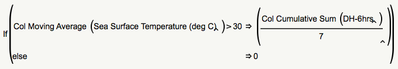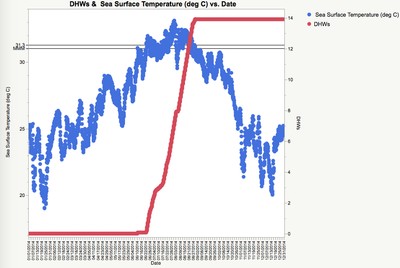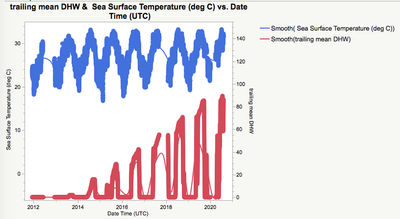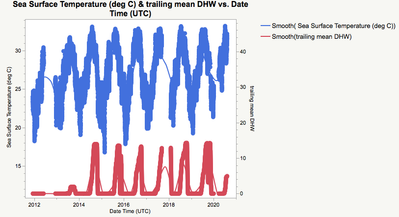Choose Language Hide Translation Bar
Highlighted

## Degree-heating weeks-help with formulas

Hello,

To understand how much heat stress corals are exposed to, we calculate what is known as a "degree-heating week:" this is the amount of time a temperature is above a known threshold (typically the mean monthly maximum or the bleaching threshold). Basically, I need to integrate the amount of time that the temperature has surpassed 31C in my dataset (attached). Temperature was measured four times/day. I can have a simple script in which every 6-hr period in which temperature is above 31C is recoded as 0.25, with <31C = 0. I could then have a trailing sum in another column and then divide by seven to convert to weeks. Basically, the DHW is only considered over the highest 3-month period over the year (typically the summer), and we know that DHW of 4 to 8 in a 3-month window results in coral bleaching.This is basically giving me what I want because, since I told it to evaluate the formula when the trailing mean was over 30C, it won't keep trying to calculate it in the winter. What I want to know is whether you can enter an argument to where each year in a multi-year dataset is analyzed individually (e.g. "by year")? I guess you could simply recreate this formula for each year (2013-2020 in this case), then subset by year, but I did want to know if you could actually do any sort of "formula reset by year" or anything like that within a single column. What I really want to do is have the formula reset to 0 after a certain period has transpired during which no additional 31C temperatures are logged, but I actually think this isn't possible!

https://coralreefwatch.noaa.gov/satellite/methodology/methodology.php#dhw

Anderson B. Mayfield
4 REPLIES 4
Highlighted

## Re: Degree-heating weeks-help with formulas

I think a figure may better explain what I want to do:so the red line represents the degree-heating weeks. Instead of plateauing, it should basically drop back to zero as temperatures fall (blue). But for the life of me, I can't think of how to script this!

Anderson B. Mayfield
Highlighted

## Re: Degree-heating weeks-help with formulas

I believe the following formula will give you what you want, creating only one column

``Col Sum( If( :Name( " Sea Surface Temperature (deg C)" ) > 31, 0.25, . ), :year ) / 7``

It calculates the DHW's for each year

Jim
Highlighted

## Re: Degree-heating weeks-help with formulas

Thanks! That is a handy formula, and I did want that information (DHW/year).

But that I really want to do is basically suppress the DHW calculation at time in which no heat is accumulating (i.e., the plateau phase).Basically, I told JMP to only calculate the DHW when temperatures were above 29 because I want the DHW to fall back to 0 once the temperature has dropped. Is it possible to define "year" in the moving average formula? In that case, I could plot DHW over month for each of the year. If I don't do that, the DHW will keep accumulating from one year to the next!

Anderson B. Mayfield
Highlighted

## Re: Degree-heating weeks-help with formulas

I think I kind of got what I wanted by playing around with the formulas. Basically, I suppressed the calculation of the DHW under 28C (since nothing interesting is happening then) and then did a trailing mean DHW over the preceding 90 days since eventually the DHW will fall to 0 once the temperature hits 28. This then means the trailing mean DHW eventually tapers off. Then, the plot effectively resets from year to year. It's kind of cheating, but in the end, it gave me what I wanted! Still, I am open to suggestions if people can think of a better, more objective way to do this that doesn't require so much arbitrary binning and tweaking.Anderson B. Mayfield
Article Labels